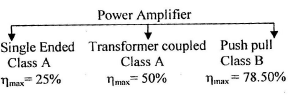Courses

# Chapter 3 Power Amplifier - Notes, Basic Electronics, Electrical Engineering Electrical Engineering (EE) Notes | EduRev

## Electrical Engineering (EE) : Chapter 3 Power Amplifier - Notes, Basic Electronics, Electrical Engineering Electrical Engineering (EE) Notes | EduRev

The document Chapter 3 Power Amplifier - Notes, Basic Electronics, Electrical Engineering Electrical Engineering (EE) Notes | EduRev is a part of the Electrical Engineering (EE) Course Electrical Engineering SSC JE (Technical).
All you need of Electrical Engineering (EE) at this link: Electrical Engineering (EE)

POWER AMPLIFIER

POWER AMPLIFIER-Large Signal Amplifier Power amplifier are meant for large signal amplification power stage is normally the final stage of a cascade amplifier and driver. The energy conversion device i.e. speaker, antenna or transducer etc. The power amplifier does not actually amplifier the power, instead it takes power from the dc power supply connected to the power circuit and converts into use full ac signal power Zin ® high. Zout ® low, heat sink are used.

Class A

Here the dc bias is adjusted much less than its cut off value so that the output current flow for full input voltage cycle.
Feature:
1. Minimum distortion.
2. Since the output contains only one frequency component, the output power is small.
3. Efficiency is low.
4. Used as voltage amplifier as modulating amplifier.

Class B

Here the dc bias is adjusted equal to the cut off value so that the output current flow for half of the input voltage cycle. Hence the output dc component will be always equal to zero.

Feature:
1. High distortion
2. High power output
3. Efficiency is large
4. Used as power amplifier as modulated amplifier.

Class AB

Here the dc bias is adjusted slightly less than is cut-off value so that the output current flows for more than half but less than input voltage cycle. This class of amplifier is just a compromise between class A and class B amplifier.

Feature:
1
. Output power is medium
2. Medium distortion
3. Medium efficiency
4. Used as modulating amplifier
5. Used with untuned power amplifier.

Class C
Here the dc bias is adjusted greater than its cutoff value so that the output current flow for less than half of the input voltage cycle.
Therefore the output current will be in the from of pulses which will be highly rich in harmonic i.e. frequency multiplier.

Feature:
1
. Very high output power due to rich in harmonic
2. Very high distortion
3. Very high conversion efficiency
4. Used as only modulated amplifier when the need is to raise the power level before transmission
5. Used with tuned frequency amplifier.Offer running on EduRev: Apply code STAYHOME200 to get INR 200 off on our premium plan EduRev Infinity!

65 docs|37 tests

,

,

,

,

,

,

,

,

,

,

,

,

,

,

,

,

,

,

,

,

,

,

,

,

,

,

,

;